# Finding Factors of a Number

Finding Factors of a Number• Factors are numbers that divide exactly into another number, which is called the multiple.
• Factors can be multiplied to make the multiple.
• 4 × 2 = 8 and so, both 4 and 2 are factors of 8.
• Factors often come in factor pairs which are the two numbers that multiply together to make the multiple.
• 4 and 2 are factor pairs for 8 because they multiply to make 8.
• 1 × 8 = 8 and so, 1 and 8 are both factor pairs of 8.
• The factors of 8 are: 1, 2, 4 and 8.

A factor is a number which divides exactly into the number that it is a factor of.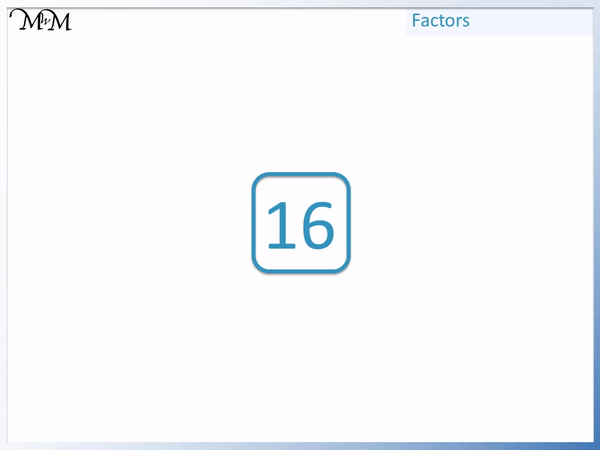• If a number is a factor of another number, it will divide into the other number exactly without remainder.
• We will find the factors of 16.
• We start with 1 because 1 is a factor of every number.
• 1 × 16 = 16 and so, 1 and 16 are factor pairs of 16.
• We now try the next number: 2.
• 2 × 8 = 16 and so, both 2 and 8 are factor pairs of 16.
• 3 does not divide exactly into 16 and so, it is not a factor.
• 4 × 4 = 16 and so 4 is a factor of 16.
• 4 pairs with itself so it does not have another number as its factor pair.

• We have arranged the numbers from smallest to largest around the sides of 16, writing each factor pair at a time.
• When the numbers in this circle meet in the middle we have found all of our factors.
• The factors of 16 are 1, 2, 4, 8 and 16Supporting Lessons#### Finding Factors Calculator

Enter the number below to find all of its factors:

# How to Find Factors of a Number

## What is a Factor of a Number

A factor is a number that divides exactly into another number.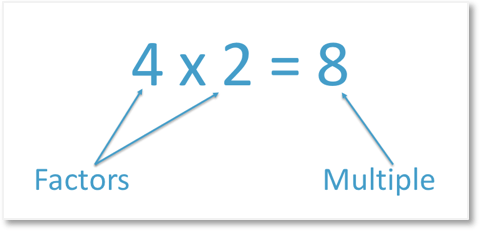The larger number that factors divide into exactly is called the multiple. Factors can be multiplied by a whole number to make this multiple.

In the example above, both 2 and 4 are factors of 8 because they can be multiplied to make 8. 8 is therefore both a multiple of 2 and a multiple of 4.

## What are Factor Pairs?

Factor pairs are two whole numbers which multiply together to make another whole number. Both numbers are factors of the number that they multiply to make. This means that they divide exactly into this number without a remainder.In the example above, both 2 and 4 are factor pairs which multiply to make 8.

All factors come in pairs except for the square root factor of square numbers.

For example, we will look at the list of all factors of 16 below.1 is a factor of every number because it divides exactly into every number. 1 is a factor pair with the number itself.

1 × 16 = 16 and so, the first factor pair of 16 is 1 and 16.

2 × 8 = 16 and so both 2 and 8 form a factor pair of 16.

4 × 4 = 16 and so 4 does not form a factor pair because it does not multiply by a different number to make 16.

All factors come in pairs except for square roots of the number itself. A square root is the number that is multiplied by itself to make the given number.

4 is the square root of 16, which means that 4 multiplied by itself makes 16. And because 4 is the square root of 16, it does not form a factor pair with another number.

Here are the factor pairs of 12.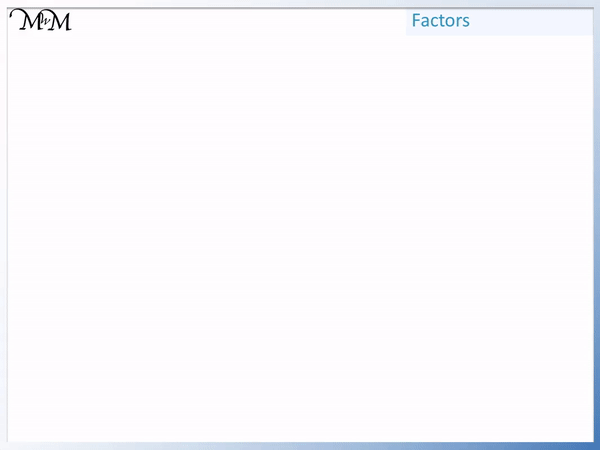6 × 2 = 12

3 × 4 = 12

1 × 12 = 12

Each of the factors of 12 come in pairs.

## How to Find Factors of a Number

To find factors of a number, use the following steps.

• Write down the number 1 and the given number at each end of a list.
• Try dividing all of the numbers that come after 1 into the larger number.
• If these numbers divide exactly into the given number with no remainder, then they are factors.
• The answer to each of these exact divisions is also a factor.
• Write down each number that divides exactly along with the answer to the division.
• Write these numbers in ascending order in the list.
• Stop when you reach the square root of the number itself or another number on the list.

When teaching finding factors of numbers, it can be useful to write the given number itself in the centre and write the factors around the outside.

In this example we will find a list of all factors of 18.

We first write down the number 1 and the given number, 18. We write 1 at one end and 18 at the other end. We will put any other factors in between.This is because 1 × 18 = 18. 1 is always a factor of every number and so is the number itself.

We will now try the numbers that come after 1, such as 2, 3, 4, 5 and so on.

2 × 9 = 18 and so, both 2 and 9 are factors of 18. We write down the 2 after the 1 in our list and we write the 9 before the 18 on our list.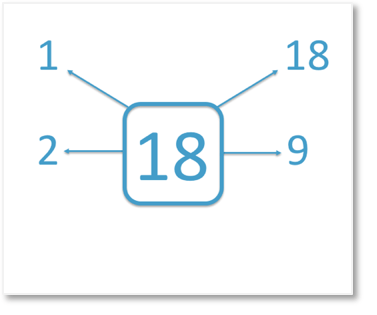9 will be the second biggest factor in our answer list after 18. We now know that no numbers between 9 and 18 will be on this list of factors.

We now try 3.

3 × 6 = 18 and so, we write down both 3 and 6 on our list of factors.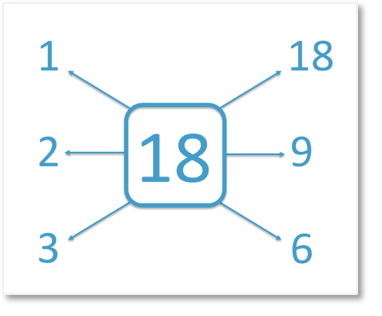We write 3 after the 2 and we write the 6 before the 9.

We now try 4.

4 does not divide exactly into 18 and so it is not a factor of 18. We do not write it down.

5 does not divide exactly into 18 either.

The next number after 5 is 6, but 6 is already on our list of factors because it paired with 3.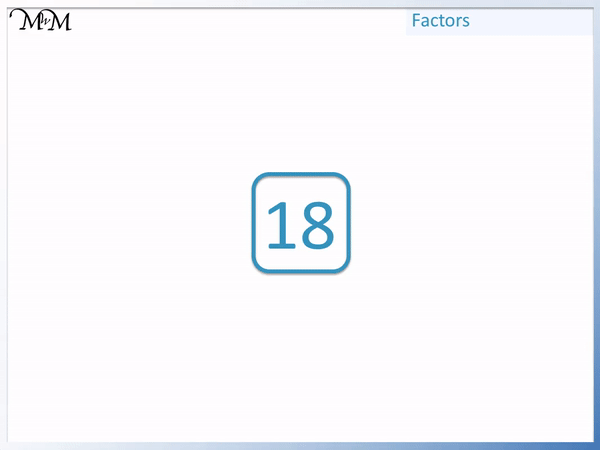Since we have now reached numbers that are already on our list we can stop as we know that we have found every factor of 18.

The factors of 18 are: 1, 2, 3, 6, 9 and 18.Now try our lesson on Multiples of Numbers where we learn how to find multiples of a number.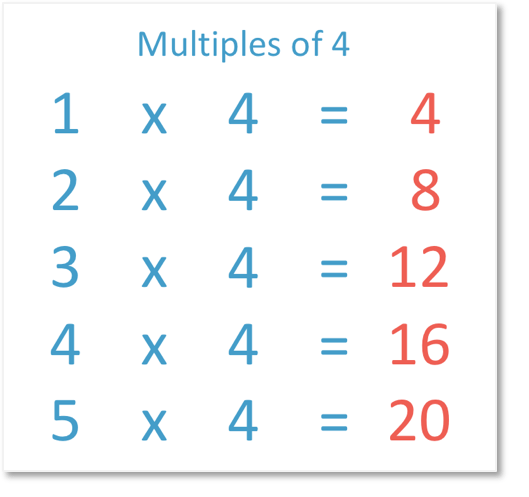error: Content is protected !!